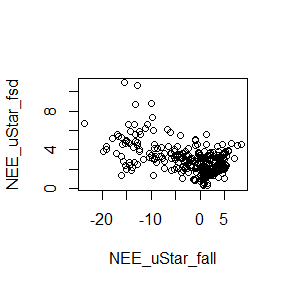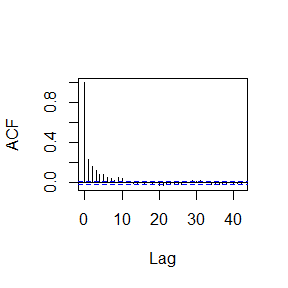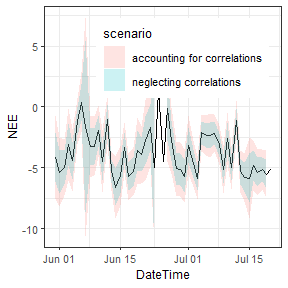# Aggregating uncertainty to daily and annual values

## Example setup

We start with half-hourly $$u_*$$-filtered and gap-filled NEE_f values. For simplicity this example uses data provided with the package and omits $$u_*$$ threshold detection but rather applies a user-specified threshold.

With option FillAll = TRUE, an uncertainty, specifically the standard deviation, of the flux is estimated for each record during gapfilling and stored in variable NEE_uStar_fsd.

library(REddyProc)
library(dplyr)
EddyDataWithPosix <- Example_DETha98 %>%
filterLongRuns("NEE") %>%
fConvertTimeToPosix('YDH',Year = 'Year',Day = 'DoY', Hour = 'Hour')
EProc <- sEddyProc$new( 'DE-Tha', EddyDataWithPosix, c('NEE','Rg','Tair','VPD', 'Ustar')) EProc$sMDSGapFillAfterUstar('NEE', uStarTh = 0.3, FillAll = TRUE)
results <- EProc$sExportResults() summary(results$NEE_uStar_fsd)
##     Min.  1st Qu.   Median     Mean  3rd Qu.     Max.
##  0.03535  1.69960  2.32796  2.74246  3.44417 24.55782

We can inspect, how the uncertainty scales with the flux magnitude.

plot( NEE_uStar_fsd ~ NEE_uStar_fall, slice(results, sample.int(nrow(results),400)))## Wrong aggregation without correlations

With neglecting correlations among records, the uncertainty of the mean annual flux is computed by adding the variances. The mean is computed by $$m = \sum{x_i}/n$$. And hence its standard deviation by $$sd(m) = \sqrt{Var(m)}= \sqrt{\sum{Var(x_i)}/n^2} = \sqrt{n \bar{\sigma^2}/n^2} = \bar{\sigma^2}/\sqrt{n}$$. This results in an approximate reduction of the average standard deviation $$\bar{\sigma^2}$$ by $$\sqrt{n}$$.

results %>% filter(NEE_uStar_fqc == 0) %>% summarise(
nRec = sum(is.finite(NEE_uStar_f))
, varSum = sum(NEE_uStar_fsd^2, na.rm = TRUE)
, seMean = sqrt(varSum) / nRec
, seMeanApprox = mean(NEE_uStar_fsd, na.rma = TRUE) / sqrt(nRec)
) %>% select(nRec, seMean, seMeanApprox)
##    nRec     seMean seMeanApprox
## 1 10901 0.02988839   0.02650074

Due to the large number of records, the estimated uncertainty is very low.

## Considering correlations

When observations are not independent of each other, the formulas now become $$Var(m) = s^2/n_{eff}$$ where $$s^2 = \frac{n_{eff}}{n(n_{eff}-1)} \sum_{i=1}^n \sigma_i^2$$, and with the number of effective observations $$n_{eff}$$ decreasing with the autocorrelation among records (Bayley 1946, Zieba 2011).

The average standard deviation $$\sqrt{\bar{\sigma^2_i}}$$ now approximately decreases only by about $$\sqrt{n_{eff}}$$:

$Var(m) = \frac{s^2}{n_{eff}} = \frac{\frac{n_{eff}}{n(n_{eff}-1)} \sum_{i=1}^n \sigma_i^2}{n_{eff}} = \frac{1}{n(n_{eff}-1)} \sum_{i=1}^n \sigma_i^2 \\ = \frac{1}{n(n_{eff}-1)} n \bar{\sigma^2_i} = \frac{\bar{\sigma^2_i}}{(n_{eff}-1)}$

First we need to quantify the error terms, i.e. model-data residuals. For all the records of good quality, we have an original measured value NEE_uStar_orig and modelled value from MDS gapfilling, NEE_uStar_fall. The residual of bad-quality data is set to missing.

results <- EProc$sExportResults() %>% mutate( resid = ifelse(NEE_uStar_fqc == 0, NEE_uStar_orig - NEE_uStar_fall, NA ) ) Now we can inspect the the autocorrelation of the errors. acf(results$resid, na.action = na.pass, main = "")The empiricical autocorrelation function shows strong positive autocorrelation in residuals up to a lag of 10 records.

Computation of effective number of observations is provided by function computeEffectiveNumObs from package lognorm based on the empirical autocorrelation function for given model-data residuals.

library(lognorm)
## Warning: package 'lognorm' was built under R version 3.5.3
autoCorr <- computeEffectiveAutoCorr(results$resid) nEff <- computeEffectiveNumObs(results$resid, na.rm = TRUE)
c( nEff = nEff, nObs = sum(is.finite(results$resid))) ## nEff nObs ## 3870.283 10901.000 We see that the effective number of observations is only about a third of the number of observations. Now we can use the formulas for the sum and the mean of correlated normally distributed variables to compute the uncertainty of the mean. results %>% filter(NEE_uStar_fqc == 0) %>% summarise( nRec = sum(is.finite(NEE_uStar_fsd)) , varMean = sum(NEE_uStar_fsd^2, na.rm = TRUE) / nRec / (!!nEff - 1) , seMean = sqrt(varMean) #, seMean2 = sqrt(mean(NEE_uStar_fsd^2, na.rm = TRUE)) / sqrt(!!nEff - 1) , seMeanApprox = mean(NEE_uStar_fsd, na.rm = TRUE) / sqrt(!!nEff - 1) ) %>% select(seMean, seMeanApprox) ## seMean seMeanApprox ## 1 0.05016727 0.04448115 ## Daily aggregation When aggregating daily respiration, the same principles hold. However, when computing the number of effective observations, we recommend using the empirical autocorrelation function estimated on longer time series of residuals (autoCorr computed above) in computeEffectiveNumObs instead of estimating them from the residuals of each day. results <- results %>% mutate( DateTime = EddyDataWithPosix$DateTime
, DoY = as.POSIXlt(DateTime - 15*60)\$yday # midnight belongs to the previous
)
aggDay <- results %>% group_by(DoY) %>%
summarise(
DateTime = first(DateTime)
, nRec = sum( NEE_uStar_fqc == 0, na.rm = TRUE)
, nEff = computeEffectiveNumObs(
resid, effAcf = !!autoCorr, na.rm = TRUE)
, NEE = mean(NEE_uStar_f, na.rm = TRUE)
, sdNEE = if (nEff == 0) NA_real_ else sqrt(
mean(NEE_uStar_fsd^2, na.rm = TRUE) / (nEff - 1))
, sdNEEuncorr = if (nRec == 0) NA_real_ else sqrt(
mean(NEE_uStar_fsd^2, na.rm = TRUE) / (nRec - 1))
)
aggDay
## # A tibble: 365 x 7
##      DoY DateTime             nRec  nEff      NEE  sdNEE sdNEEuncorr
##    <int> <dttm>              <int> <dbl>    <dbl>  <dbl>       <dbl>
##  1     0 1998-01-01 00:30:00    21  7.87  0.124    0.988       0.579
##  2     1 1998-01-02 00:30:00     7  3.66  0.00610  1.57        1.05
##  3     2 1998-01-03 00:30:00     0  0     0.0484  NA          NA
##  4     3 1998-01-04 00:30:00     0  0     0.303   NA          NA
##  5     4 1998-01-05 00:30:00    28 10.9   0.195    0.851       0.515
##  6     5 1998-01-06 00:30:00    48 18.0   0.926    0.615       0.370
##  7     6 1998-01-07 00:30:00    48 18.0  -0.337    0.566       0.340
##  8     7 1998-01-08 00:30:00    46 17.2  -0.139    0.541       0.325
##  9     8 1998-01-09 00:30:00    45 16.8   0.614    0.482       0.289
## 10     9 1998-01-10 00:30:00    36 13.5   0.242    0.646       0.386
## # ... with 355 more rowsThe confidence bounds (+-1.96 stdDev) computed with accounting for correlations in this case are about twice the ones computed with neglecting correlations.